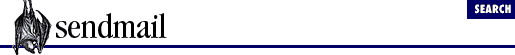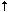home | O'Reilly's CD bookshelfs | FreeBSD | Linux | Cisco | Cisco Exam

#Chapter 6The Mail Hub and Delivery Agents## 6.4 Add the Missing Parts to Mhub

The final step in creating the ``` hub``` mail delivery agent definition is to fill in its missing ``` F=``` , ``` S=``` , ``` R=``` , and ``` T=``` equates.

Edit the client.cf file again and add the new parts shown below to the ``` Mhub``` definition:

```# Delivery agent definition to forward mail to hub
Mhub,   P=[IPC], ```

S=0, R=0, F=mDFMuXa
```
, ```

T=DNS/RFC822/SMTP
```
, A=IPC \$hnew  new  new        new
```

Here, the ``` S=``` and ``` R=``` equates are given a value of zero. The ``` S=``` equate specifies the sender-rewriting rule set. The ``` R=``` equate specifies the recipient-rewriting rule set. Because there are no rule sets yet, these equates are set to zero. You will be giving them real rule-set numbers when we begin to cover rule sets in Chapter 8 .

The ``` T=``` is the same as the ``` T=``` in ``` local``` except that instead of expecting UNIX errors, we will expect SMTP errors because we will be speaking SMTP to the hub. More about this later.

The flags listed in the ``` F=mDFMuXa``` equate of the ``` hub``` definition are typical of those generally used in ``` [IPC]``` delivery agent definitions. You may want to change these depending on your needs. All the available flags are listed in Chapter 30 . The ones we selected are summarized in Table 6.1 .

Table 6.1: The hub Delivery Agent's F= Flags
Flag Description
m This agent can deliver to more than one user at a time.
D Include a ``` Date:``` in the header (if one is not present).
F Include a ``` From:``` in the header (if one is not present).
M Include a ``` Message-ID:``` in the header (if one is not present).
u Preserve the case of the recipient name.
X Pass lone dots on a line by doubling them.
a Run extended SMTP protocol

The mailer delivery agent definitions are now roughed out. Remember that the symbolic name (``` hub``` or ``` local``` ) is the only part of these definitions that will be referenced in later rule sets. Also notice that the last equate of each definition, the ``` A=``` command-line equate, ends with a macro (``` \$h``` or ``` \$u``` ). We cover macros in the next chapter.6.3 The local Delivery Agent6.5 Things to Try